# Basic Postulates and Theorem of Boolean Algebra

## Class 12 - APC Understanding ISC Computer Science with BlueJ

#### Question 1

What are the fundamental concepts of boolean algebra?

The fundamental concept of boolean algebra is to deal with logical problems in mathematics by using only two values i.e. digits 0 (zero) and 1 (one) or 'False' and 'True' or 'ON' and 'OFF' logical states.

#### Question 2

What is truth table? Explain with reference to boolean algebra.

Truth Table is the tabular representation of the values given and the result obtained due to any logical operation. For example:

1st Value2nd ValueOutput
001
010
101
110
1st Value2nd ValueOutput
FALSEFALSETRUE
FALSETRUEFALSE
TRUEFALSETRUE
TRUETRUEFALSE

#### Question 3

What do you mean by binary valued quantities?

The digits 0 (zero) and 1 (one) of the binary number system used in boolean algebra are called binary valued quantities.

#### Question 4

Draw the truth table for the following logical operators:

(a) AND logic

ABA.B
000
010
100
111

(b) OR logic

ABA+B
000
011
101
111

(c) NOT logic

AA'
01
10

#### Question 5

Find the complement of the following expressions:

(a) (A + B).(B + C).(A + C)

Complement of (A + B).(B + C).(A + C)
= [(A + B).(B + C).(A + C)]'
= (A + B)' + [(B + C).(A + C)]'
= (A + B)' + (B + C)' + (A + C)'
= A'.B' + B'.C' + A'.C'

(c) A.B + (A'.B').(B.C + B'.C')

Complement of A.B + (A'.B').(B.C + B'.C')
= [A.B + (A'.B').(B.C + B'.C')]'
= (A.B)'.[(A'.B').(B.C + B'.C')]'
= (A' + B').[(A'.B')' + (B.C + B'.C')']
= (A' + B').[A + B + [(B.C)' . (B'.C')']]
= (A' + B').[A + B + [(B' + C').(B + C)]]
= (A' + B').[A + B + [BB' + B'C + BC' + CC']]
= (A' + B').[A + B + [0 + B'C + BC' + 0]]
= (A' + B').(A + B + B'C + BC')
= (A' + B').(A + B'C + B(1 + C'))
= (A' + B').(A + B + B'C)

#### Question 6

What do you understand by Karnaugh's map? Explain.

Karnaugh's map is a way to reduce an expression by using a tabular or matrix representation to its most simplified form. It is named after its inventor Maurice Karnaugh. Its advantage is that it doesn't require any algebraic derivation. It has a limitation that it will reduce the expression only when it is in canonical SOP or POS form.

#### Question 7

Reduce the following boolean functions with the help of Karnaugh's map:

(a) F (a, b, c, d) = Σ (1, 2, 3, 11, 12, 14, 15)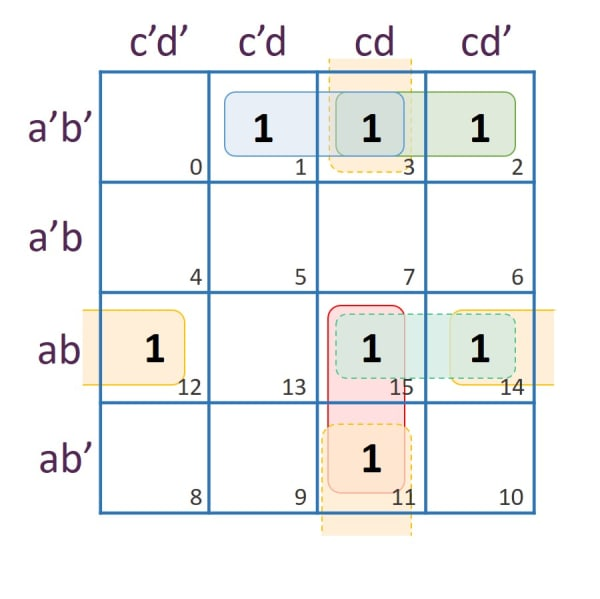From Pair (1,3):
Rows representing the pair: a'b'
Columns representing the pair: c'd + cd = d
Term Obtained = a'b'd

From Pair (3,2):
Rows representing the pair: a'b'
Columns representing the pair: cd + cd' = c
Term Obtained = a'b'c

From Pair (12,14):
Rows representing the pair: ab
Columns representing the pair: c'd' + cd' = d'
Term Obtained = abd'

From Pair (15,11):
Rows representing the pair: ab + ab' = a
Columns representing the pair: cd
Term Obtained = acd

Pairs (15,14) and (3,11) are redundant

Simplified expression = a'b'd + a'b'c + abd' + acd

(b) F (A, B, C, D) =Σ (0, 1, 2, 3, 12, 13, 14, 15)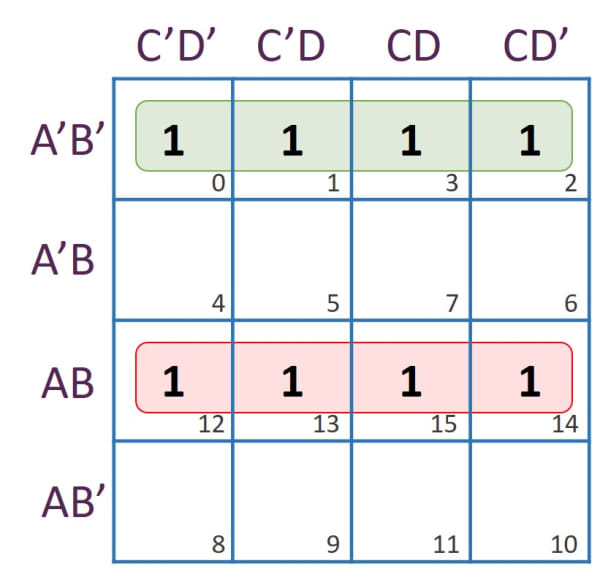Columns representing the quad: 1 (Both variables C and D are in opposite form. Hence, they get cancelled.)
Term Obtained = A'B'

Columns representing the quad: 1 (Both variables C and D are in opposite form. Hence, they get cancelled.)
Term Obtained = AB

Simplified expression = AB + A'B'

(c) F (a, b, c, d) =Σ (0, 1, 2, 4, 5, 6, 8, 9, 12, 13, 14)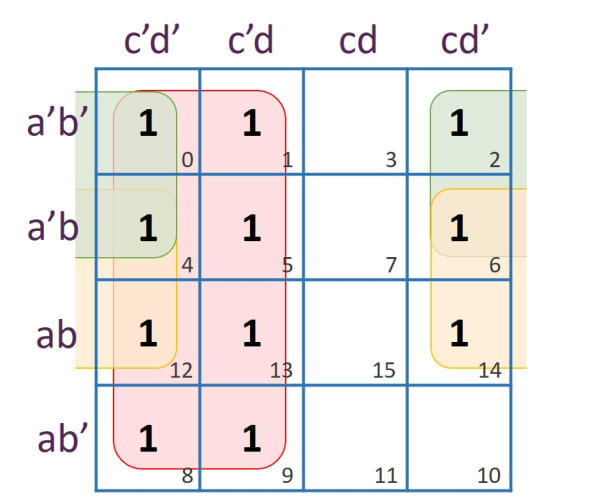From Octet (0,1,4,5,12,13,8,9):
Rows representing the Octet: 1 (Both variables a and b are in opposite form. Hence, they get cancelled.)
Columns representing the Octet: c'd' + c'd = c'
Term Obtained = c'

Rows representing the quad: a'b' + a'b = a'
Columns representing the quad: c'd' + cd' = d'
Term Obtained = a'd'

Rows representing the quad: a'b + ab = b
Columns representing the quad: c'd' + cd' = d'
Term Obtained = bd'

Simplified expression = c' + a'd' + bd'

#### Question 8

Write max term expression corresponding to the following truth table:

ABCD (Output)
0000
0010
0100
0111
1000
1011
1101
1111

ABCD
(Output)
Max
Terms
Max Term
Designation
0000A+B+CM0
0010A+B+C'M1
0100A+B'+CM2
0111
1000A'+B+CM4
1011
1101
1111

Max term expression for D = (A+B+C).(A+B+C').(A+B'+C).(A'+B+C)

Max term expression in cardinal form:
F(A, B, C) = π(0, 1, 2, 4)

#### Question 9

Draw the truth table for the logical function M for three inputs A, B and C, where M = F (A, B, C). The output is 0 (zero), if the majority of inputs are zero (0) and one (1) if the majority of inputs are one (1). Write the sum of products expression (SOP) for M.

ABCOutputMin Terms
0000
0010
0100
0111A'BC
1000
1011AB'C
1101ABC'
1111ABC

SOP expression for F (A, B, C):
A'BC + AB'C + ABC' + ABC

#### Question 10

Draw the truth table for the following boolean function:
F (A, B, C)=(A'+B).(B'+C)

ABCA'B'A'+BB'+C(A'+B).(B'+C)
00011111
00111111
01010100
01110111
10001010
10101010
11000100
11100111

#### Question 11

Write the SOP expression corresponding to the following truth table:

ABCD (Output)
0000
0011
0100
0111
1000
1011
1100
1111

ABCD
(Output)
Min
Terms
0000
0011A'B'C
0100
0111A'BC
1000
1011AB'C
1100
1111ABC

SOP expression:
A'B'C + A'BC + AB'C + ABC

#### Question 12

Express in the product of sum (POS) form, the boolean function F (A, B, C). The truth table for which is given below:

ABCF
0000
0011
0100
0111
1000
1011
1100
1111

ABCFMax
Terms
0000A+B+C
0011
0100A+B'+C
0111
1000A'+B+C
1011
1100A'+B'+C
1111

SOP expression:
(A+B+C).(A+B'+C).(A'+B+C).(A'+B'+C)

#### Question 14

(a) Find the complement of XY'Z + XY + YZ'

Complement of XY'Z + XY + YZ'
= [XY'Z + XY + YZ']'
= (XY'Z)'.(XY)'.(YZ')'
= (X' + Y + Z').(X' + Y').(Y' + Z)

(b) Convert the following expression into canonical POS form:
F(A, B) = (A + B).A'

(A + B).(A' + BB')
= (A + B).(A' + B).(A' + B')

#### Question 15

Minimize the following function by using K-Map:
F(A, B, C) = A'BC' + A'BC + ABC' + ABC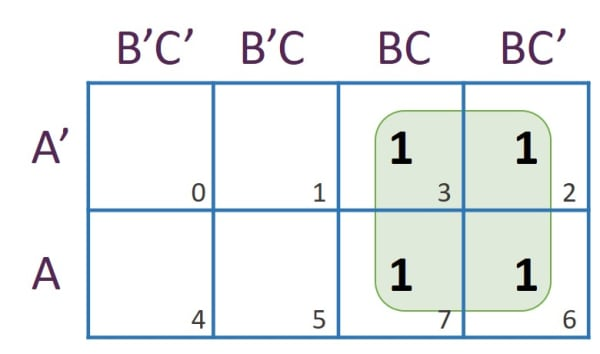Rows representing the quad: A + A' = 1
Columns representing the quad: BC + BC' = B
Term Obtained = B

Simplified expression:
F(A, B, C) = B

#### Question 16

Reduce the following function by using Boolean laws:
F(A, B, C, D) = (A' + C) (A' + C') (A' + B + C'D)

``````
(A' + C) (A' + C') (A' + B + C'D)
= (A'A' + A'C' + CA' + CC')(A' + B + C'D) [Distributive Law]
= (A' + A'C' + A'C + 0)(A' + B + C'D)     [∵ CC' = 0, A'A' = A']
= [A'(1 + C' + C)](A' + B + C'D)
= [A'(1 + C)](A' + B + C'D)               [∵ 1 + C' = 1]
= A'(A' + B + C'D)                        [∵ 1 + C = 1]
= (A'A' + A'B + A'C'D)                    [Distributive Law]
= (A' + A'B + A'C'D)                      [∵ A'A' = A']
= [A'(1 + B) + A'C'D]
= [A' + A'C'D]                            [∵ 1 + B = 1]
= [A'(1 + C'D)]                           [∵ 1 + C'D = 1]
= A'
```
```

#### Question 17

State the principal of duality. Write the dual of:
(P + Q').R.1 = P.R + Q'.R

A boolean expression can be converted into another form by replacing each plus(+) sign with a dot(.) and each dot sign with a plus, each 1 with a 0 and each 0 with a 1. The expression so obtained is known as dual of the given boolean expression and the process of conversion is termed as Principle of Duality.

Dual of (P + Q').R.1 = P.R + Q'.R is:
(P.Q') + R + 0 = (P + R).(Q' + R)

#### Question 18

Minimize the expression using Boolean laws:
(A + B')(B + CD)'

``````
(A + B')(B + CD)'
= (A + B')(B'(CD)')                 [De-Morgan's Law]
= (A + B')(B'(C' + D'))             [De-Morgan's Law]
= (A + B')(B'C' + B'D')             [Distributive Law]
= AB'C' + AB'D' + B'B'C' + B'B'D'
= AB'C' + AB'D' + B'C' + B'D'       [∵ B'B' = B']
= B'C'(1 + A) + B'D'(1 + A)
= B'C' + B'D'                       [∵ 1 + A = 1]
```
```

#### Question 19

Convert the following cardinal form of expression into canonical form:
F(P, Q, R) = π(1, 3)

Binary of 1 is 001: P + Q + R'
Binary of 3 is 011: P + Q' + R'

Canonical form:
(P + Q + R').(P + Q' + R')

#### Question 20

In the following truth table x and y are inputs and B and D are outputs:

InputOutput
xyBD
0000
0111
1001
1100

(i) Write the SOP expression for D.

xyDMin
Terms
000
011x'y
101xy'
110

SOP expression = x'y + xy'

(ii) Write the POS expression for B

xyBMax
Terms
000x+y
011
100x'+y
110x'+y'

POS expression = (x+y).(x'+y).(x'+y')

#### Question 21

A combinational logic circuit with three inputs P,Q,R produces 1 if and only if an odd number of 0's are inputs.

1. Draw a truth table.
2. Derive a canonical SOP expression for the above truth table.
3. Find the complement of the above derived expression using De-Morgan's theorem and verify whether it is equivalent to its POS expression or not.

Truth Table

PQROutputMin
Terms
Max
Terms
0001P'Q'R'
0010P+Q+R'
0100P+Q'+R
0111P'QR
1000P'+Q+R
1011PQ'R
1101PQR'
1110P'+Q'+R'

SOP expression = P'Q'R' + P'QR + PQ'R + PQR'

POS expression = (P+Q+R').(P+Q'+R).(P'+Q+R).(P'+Q'+R')

Complement of P'Q'R' + P'QR + PQ'R + PQR'
= [P'Q'R' + P'QR + PQ'R + PQR']'
= (P'Q'R')'.(P'QR)'.(PQ'R)'.(PQR')'
= (P''+Q''+R'').(P''+Q'+R').(P'+Q''+R').(P'+Q'+R'')
= (P+Q+R).(P+Q'+R').(P'+Q+R').(P'+Q'+R)

#### Question 22.

Using a truth table, verify the following expression:
X + (Y + Z) = (X + Y) + Z

XYZY+ZX+(Y+Z)(X+Y)(X+Y)+Z
0000000
0011101
0101111
0111111
1000111
1011111
1101111
1111111

As the values of columns X+(Y+Z) and (X+Y)+Z are identical, hence the expression is proved.

#### Question 23

Given F (X, Y, Z) = (X' + Y').(Y + Z')
Write the function in canonical POS form.

Expression in canonical POS form:
(X' + Y' + ZZ').(XX' + Y + Z')
= (X' + Y' + Z).(X' + Y' + Z').(X + Y + Z').(X' + Y + Z')

#### Question 24

Find the complement of the following expression:
X' + XY'

Complement of X' + XY':

``````

= [X' + XY']'
= X''.(XY')'    [De-Morgan's Law]
= X.(X' + Y'')  [De-Morgan's Law]
= X.(X' + Y)
= X.X' + X.Y    [Distributive Law]
= 0 + X.Y       [∵ X.X' = 0]
= X.Y

```
```

#### Question 26

State the two absorption laws. Verify any one of them using truth table.

1. A + A.B = A
2. A.(A + B) = A

Proof of First Law:

By using algebraic method

L.H.S = A + A.B
= A(1 + B)
= A.1
= A
L.H.S = R.H.S
Hence proved.

By using truth table

ABA.BA+A.B
0000
0100
1001
1111

The columns A and A+A.B have same values, hence proved.

#### Question 27

If A = 1, B = 0, C = 1 and D = 1, find its

1. maxterm
2. minterm

Maxterm:
A' + B + C' + D'

Minterm:
A . B' . C . D

#### Question 28

Give the dual of the following:
(A' B) + (C' 1) = (A' + C) (B + C)

Dual of (A' B) + (C' 1) = (A' + C) (B + C) is:
(A' + B).(C' + 0) = (A'.C) + (B.C)

#### Question 29

Reduce the following Boolean expression into their simplest forms:

1. {(CD)' + A} + A + C.D + A.B
2. A.{B + C (A.B + A.C)'}

{(CD)' + A} + A + C.D + A.B

``````
{(CD)' + A} + A + C.D + A.B
= C' + D' + A + A + C.D + A.B           [De-Morgan's Law]
= C' + D' + A + C.D + A.B               [∵ A+A=A]
= A(1 + B) + C' + D' + C.D
= A + C' + D' + C.D                     [∵ 1+B=1]
= (A + C' + D' + C).(A + C' + D' + D)   [Distributive Law]
= (A + D' + 1).(A + C' + 1)             [∵ C'+C=1, D'+D=1]
= 1.1                                   [∵ A+D'+1=1, A+C'+1=1]
= 1
```
```

A.{B + C (A.B + A.C)'}

``````
A.{B + C(A.B + A.C)'}
A.{B + C[(A.B)'.(A.C)']}                [De-Morgan's Law]
A.{B + C[(A' + B').(A' + C')]}          [De-Morgan's Law]
A.{B + C[A'A' + A'C' + A'B' + B'C']}    [Distributive Law]
A.{B + C[A' + A'C' + A'B' + B'C']}      [∵ A'A'=A']
A.{B + C[A'(1 + C' + B') + B'C']}
A.{B + C[A'.1 + B'C']}                  [∵ 1 + C' + B' = 1]
A.{B + A'C + B'CC'}                     [Distributive Law]
A.{B + A'C + 0}                         [∵ CC'=0]
AB + AA'C                               [Distributive Law]
AB                                      [∵ AA'C=0]
```
```

#### Question 30

Convert the following cardinal expression into its canonical form and reduce it using Boolean laws:

F(L, M, O, P) = π(0, 2, 8, 10)

Binary of 0 is 0000: L+M+O+P
Binary of 2 is 0010: L+M+O'+P Binary of 8 is 1000: L'+M+O+P
Binary of 10 is 1010: L'+M+O'+P

Canonical form:
(L+M+O+P).(L+M+O'+P).(L'+M+O+P).(L'+M+O'+P)

Reducing the expression using boolean laws:

``````

(L+M+O+P).(L+M+O'+P).(L'+M+O+P).(L'+M+O'+P)
= (L+M+O+P).(L'+M+O+P).(L+M+O'+P).(L'+M+O'+P)   [Associative Law]
= [(M+O+P) + (LL')].[(M+O'+P) + (LL')]          [Distributive Law]
= [(M+O+P) + 0].[(M+O'+P) + 0]                  [Complementary Law]
= (M+O+P).(M+O'+P)                              [∵ a+0=a]
= (M+P) + (O.O')                                [Distributive Law]
= M+P+0                                         [Complementary Law]
= M+P                                           [∵ a+0=a]

```
```

#### Question 31

Prove the following Demorgan's laws using laws of boolean algebra:

(a) (A + B)' = A'.B'
(b) (A.B)' = A' + B'

The sum of a variable and its complement is 1 and their product is 0 — A+A'=1 and A.A'=0. The theorem states that A'.B' is the complement of A+B. To prove, it must satisfy:

1. (A + B)(A' . B') = 0 and
2. (A + B) + (A' . B') = 1

Consider the first case:
(A + B)(A' . B') = 0

``````
L.H.S = (A + B)(A' . B')
= A.A'.B' + B.A'.B'   [Distributive Law]
= 0.B' + 0.A'         [∵ A.A'=0 and B.B'=0]
= 0 + 0
= 0
L.H.S = R.H.S
Hence proved.
```
```

Second Case:
(A + B) + (A' . B') = 1

``````
L.H.S = (A + B) + (A' . B')
= (A + B + A')(A + B + B')    [Distributive Law]
= (1 + B)(1 + A)              [A+A'=1 and B+B'=1]
= 1.1
= 1
L.H.S = R.H.S
```
```

#### Question 32

Obtain a simplified expression for the given boolean function using Karnaugh's map:
F (a, b, c, d) = Σ(1, 2, 3, 11, 12, 14, 15)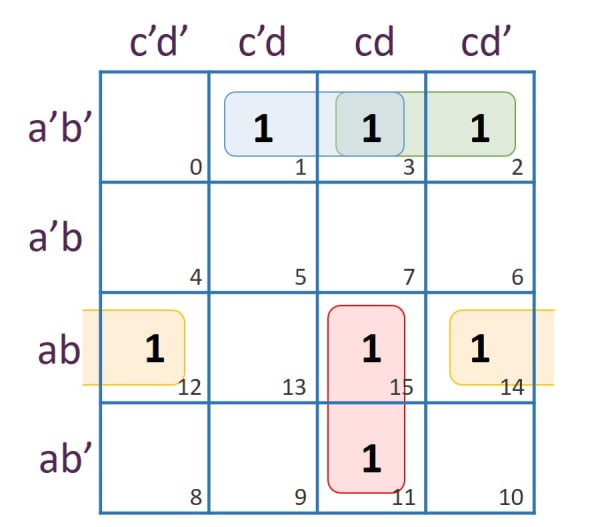From pair (1,3):
Rows representing the pair: a'b'
Columns representing the pair: c'd + cd = d
Term Obtained = a'b'd

From pair (3,2):
Rows representing the pair: a'b'
Columns representing the pair: cd + cd' = c
Term Obtained = a'b'c

From pair (12,14):
Rows representing the pair: ab
Columns representing the pair: c'd' + cd' = d'
Term Obtained = abd'

From pair (15,11):
Rows representing the pair: ab + ab' = a
Columns representing the pair: cd
Term Obtained = acd

Simplified expression:
F(a, b, c, d) = a'b'd + a'b'c + abd' + acd

#### Question 33

Reduce the following boolean functions with the help of Karnaugh's map:
F(U, V, W, Z)=Σ(0, 1, 2, 3, 12, 13, 14, 15)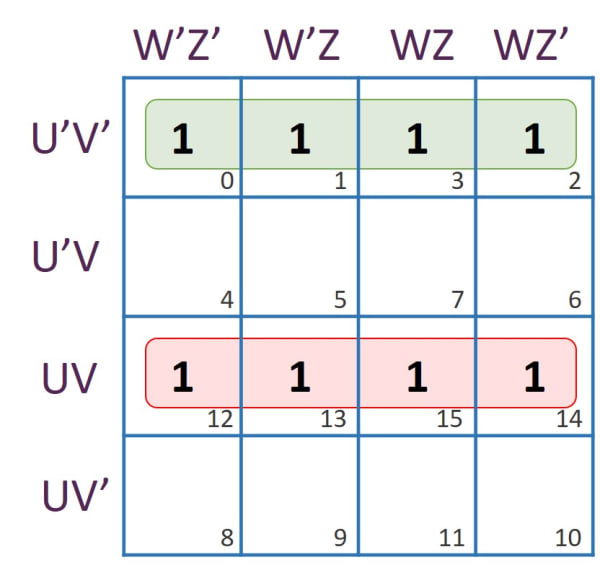Columns representing the quad: 1 (Both variables W and Z are in opposite form. Hence, they get cancelled.)
Term Obtained = U'V'

Columns representing the quad: 1 (Both variables W and Z are in opposite form. Hence, they get cancelled.)
Term Obtained = UV

Simplified expression = UV + U'V'

#### Question 34

Given: F(x, y, z)=Σ(1, 4, 5, 6, 7).
Prove that: F(x, y, z)=π(0, 2, 3).

Reducing F(x, y, z)=Σ(1, 4, 5, 6, 7) using K-Maps: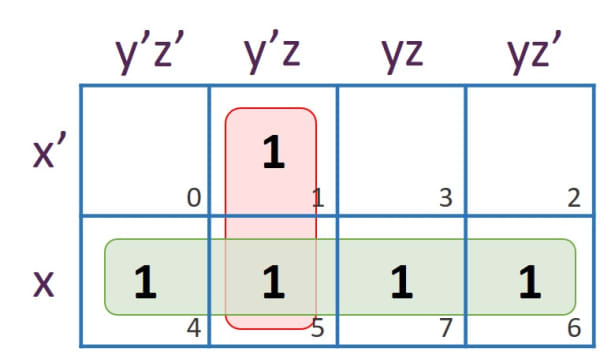From Pair (1,5):
Rows representing the Pair: x' + x = 1
Columns representing the Pair: y'z
Term Obtained = y'z

Columns representing the Quad: 1 (Both variables y and z are in opposite form. Hence, they get cancelled.)
Term Obtained = x

Result = x + y'z

Reducing F(x, y, z)=π (0, 2, 3) using K-Maps: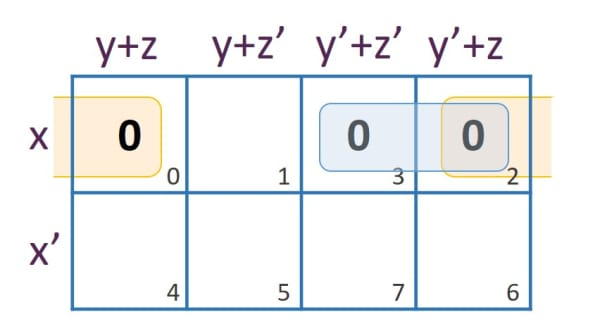From Pair (0,2):
Rows representing the Pair: x
Columns representing the Pair: (y+z).(y'+z) = z
Term Obtained = x+z

From Pair (3,2):
Rows representing the Pair: x
Columns representing the Pair: (y'+z').(y'+z) = y'
Term Obtained = x+y'

Result = (x+z).(x+y')
Reducing it further:
(x+z).(x+y')
= x.x + xy' + xz + y'z
= x(1 + y' + z) + y'z
= x.1 + y'z
= x + y'z

As both, F(x, y, z)=Σ(1, 4, 5, 6, 7) and F(x, y, z)=π(0, 2, 3) reduce to x + y'z, hence proved.

#### Question 35

State the two Idempotent laws of boolean algebra. Verify any one of them using the truth table.

This law states that when a variable is either added or multiplied to the same variable will result in the same variable.
A + A = A
A . A = A

Truth Table

AAA+A
000
111
AAA.A
000
111

#### Question 36

Given the boolean function F(x, y, z)=Σ(0, 2, 4, 5, 6). Reduce it using Karnaugh's map.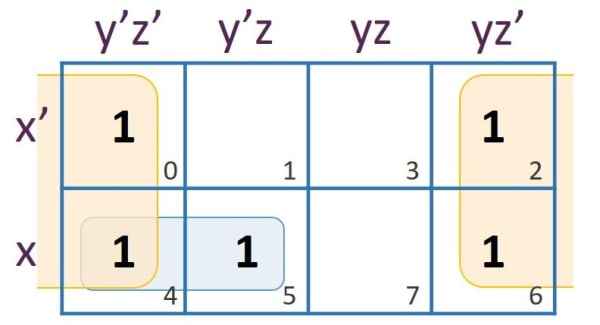Rows representing the Quad: x' + x = 1
Columns representing the Quad: y'z' + yz' = z'
Term Obtained = z'

From Pair (4,5):
Rows representing the Pair: x
Columns representing the Pair: y'z' + y'z = y'
Term Obtained = xy'

Result = xy' + z'

#### Question 37

State the dual form of the following boolean expression:
X.Y'(X.Y'.Z + X + X'.Z')

Dual of X.Y'(X.Y'.Z + X + X'.Z') is:
X+Y'+((X+Y'+Z).X.(X'+Z'))

#### Question 38

What is the application of boolean algebra in computer science?

Computers understand machine language which is based on binary logic i.e. 0's and 1's. Their eletrical circuits are a physical manifestation of two-value Boolean logic. The processors of the computer work on boolean algebra.

#### Question 39

Reduce the following to its simplest form using laws of boolean algebra. At each step clearly state the law used for simplification.
A.B' + A'.B.C' + (A.C') + B.C

``````
A.B' + A'.B.C' + (A.C') + B.C
= A.B' + A'.B.C' + (A.C')(B+B') + B.C       [Complementary Law: B+B'=1]
= A.B' + A'.B.C' + A.B.C' + A.B'.C' + B.C   [Distributive Law]
= A.B' + A.B'.C' + A'.B.C' + A.B.C' + B.C   [Associative Law]
= A.B' + A'.B.C' + A.B.C' + B.C             [Absorbtion Law: A.B' + A.B'.C' = A.B']
= A.B' + B.C + B.C'(A' + A)
= A.B' + B.C + B.C'.1                       [Complementary Law: A+A'=1]
= A.B' + B(C + C')
= A.B' + B                                  [Complementary Law: C+C'=1]
= (A + B).(B' + B)                          [Distributive Law]
= A + B                                     [Complementary Law: B+B'=1]
```
```

#### Question 40

Explain the following:

1. Canonical sum of product
2. Canonical product of sum

Canonical sum of product(SOP):
This type of expression is formed by adding the product terms of the variables. For example, A + B can be written as A.1 + B.1 and A + BC can be written as A.1 + B.C
In the first example above, A.1 and B.1 are the two product terms which are added to form the given expression. Similarly, in the next example, A.1 and B.C are added to form the expression. Hence, they are sum of product expressions.

Canonical product of sum(POS):
This type of expression is formed by the product of the sum terms of the variables. For example, A.B can be written as (A + 0).(B + 0) and A.(B + C) can be written as (A + 0).(B + C).
In the first example mentioned above, A + 0 and B + 0 are the two sum terms which are multiplied together to form the expression. Similarly, in the next example, A + 0 and B + C are multiplied to form the given expression. Hence, they are product of sum expressions.

#### Question 41

Simplify a.b + a'.c + b.c using the laws of boolean algebra. At each step, state clearly the law used for simplification.

``````
a.b + a'.c + b.c
= a.b + a'.c + b.c(a + a')      [Complementary Law: A+A'=1]
= a.b + a'.c + a.b.c + a'.b.c   [Distributive Law]
= a.b + a.b.c + a'.c + a'.b.c   [Associative Law]
= a.b + a'.c                    [Absorbtion Law]
```
```

#### Question 42

Simplify the following expression using laws of boolean algebra:
(a.b + x + y + z).(a.b + x'.y'.z')

``````
(a.b + x + y + z).(a.b + x'.y'.z')
= a.b + [(x + y + z).(x'.y'.z')]      [Distributive Law]
= a.b + [(x + y + z).(x + y + z)']    [De-Morgan's Law]
= a.b + 0                             [Complementary Law: (x + y + z).(x + y + z)' = 0]
= a.b
```
```

#### Question 43

Reduce the following boolean expression to its simple form:
A.[B + C.(A.B + A.C')]

``````
A.[B + C.(A.B + A.C')]
= A.[B + A.B.C + A.C'.C]  [Distributive Law]
= A.[B + A.B.C + 0]       [Complementary Law: C'.C = 0]
= A.[B(1 + AC)]           [Distributive Law]
= A.B                     [∵ 1+AC=1]
```
```

#### Question 44

Convert (X' + Y + Z').(X + Y' + Z).(X + Y + Z').(X + Y + Z) into SOP form.

Converting to max term designation:

Binary Pattern of max term (X' + Y + Z') = 101
Decimal Equivalent of 101 = 5
Max term designation of (X' + Y + Z') = M5

Binary Pattern of max term (X + Y' + Z) = 010
Decimal Equivalent of 010 = 2
Max term designation of (X + Y' + Z) = M2

Binary Pattern of max term (X + Y + Z') = 001
Decimal Equivalent of 001 = 1
Max term designation of (X + Y + Z') = M1

Binary Pattern of max term (X + Y + Z) = 000
Decimal Equivalent of 000 = 0
Max term designation of (X + Y + Z) = M0

Max term designation = M0, M1, M2, M5
Min term designation = m3, m4, m6, m7

m3 = 011 = X'YZ
m4 = 100 = XY'Z'
m6 = 110 = XYZ'
m7 = 111 = XYZ

SOP Expression = X'YZ + XY'Z' + XYZ' + XYZ

#### Question 45

Find the complement of F (a, b, c, d) using Demorgan's Laws. Show the relevant reasoning.
F(a, b, c, d)=a + {(b + c).(b' + d')}

``````
[a + {(b + c).(b' + d')}]'
= a'.[{(b + c).(b' + d')}]'   [De-Morgan's Law]
= a'.{(b + c)' + (b' + d')'}  [De-Morgan's Law]
= a'.{(b'c') + (b''.d'')}     [De-Morgan's Law]
= a'.{(b'c') + (bd)}          [Involution Law: a''=a]
= a'b'c' + a'bd
```
```

#### Question 46

Given the function F (a, b, c) = Σ(0, 2, 3, 4, 6). Reduce it using Karnaugh's map.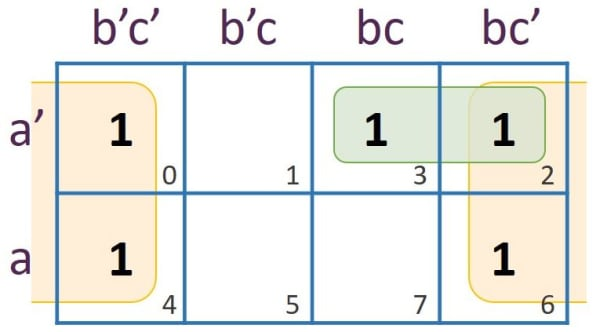Rows representing the Quad: a' + a = 1
Columns representing the Quad: b'c' + bc' = c'
Term Obtained = c'

From Pair (3,2):
Rows representing the Pair: a'
Columns representing the Pair: bc + bc' = b
Term Obtained = a'b

Result = a'b + c'

#### Question 47

State the two absorption laws of boolean algebra. Verify any one of them using the truth table.

The two absorption laws are:

1. A + A.B = A
2. A.(A + B) = A

Proof of A + A.B = A using truth table:

ABA.BA+A.B
0000
0100
1001
1111

#### Question 48

A factory needs a minimum of 1200 tons of raw material and at least 100 workers to start its production. There are three suppliers each agreed to supply 600, 800 and 1250 tons of raw materials respectively.
A=1 if the first supplier supplies else it is 0.
B=1 if the second supplier supplies else it is 0.
C=1 if the third supplier supplies else it is 0.
D=1 if 100 workers are available else it is 0.
R=1 if production starts else it is 0.
(a) Taking A, B, C and D as inputs and R as output draw truth table for the problem stated above and derive its SOP expression.
(b) Reduce the above SOP expression using the K-map.

ABCDRMin
Terms
00000
00010
00100
00111A'B'CD
01000
01010
01100
01111A'BCD
10000
10010
10100
10111AB'CD
11000
11011ABC'D
11100
11111ABCD

SOP expression:
A'B'CD + A'BCD + AB'CD + ABC'D + ABCD

K-Map to reduce the expression: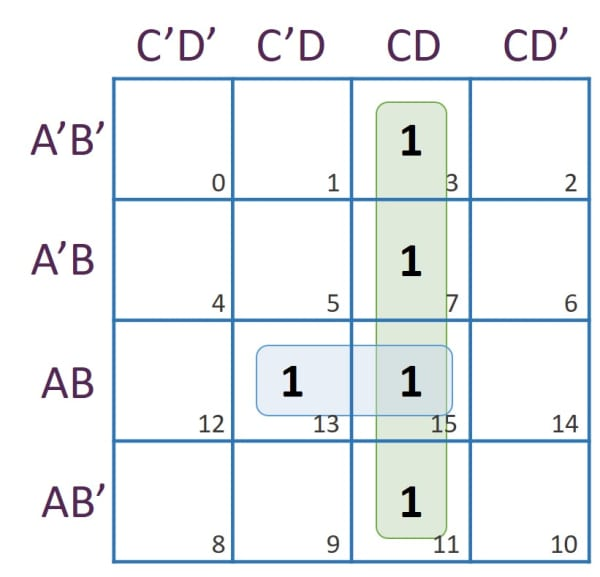Rows representing the Quad: 1 (Both variables A and B are in opposite form. Hence, they get cancelled.)
Term Obtained = CD

From Pair (13,15):
Rows representing the Pair: AB
Columns representing the Pair: C'D + CD = D
Term Obtained = ABD

Result = ABD + CD

#### Question 49

Given below is the truth table for a combinational circuit for which the input is a 3 bit number and output is its 2's complement.

InputsOutputs
XYZPQR
000000
001111
010110
011101
100100
101011
110010
111001

Write SOP expression for the outputs P, Q and R. Reduce them, if possible, using the Karnaugh's map.

Min Terms
PQR

X'Y'ZX'Y'ZX'Y'Z
X'YZ'X'YZ'
X'YZ X'YZ
XY'Z'
XY'ZXY'Z
XYZ'
XYZ

SOP for P:
X'Y'Z + X'YZ' + X'YZ + XY'Z'

SOP for Q:
X'Y'Z + X'YZ' + XY'Z + XYZ'

SOP for R:
X'Y'Z + X'YZ + XY'Z + XYZ

K-Map for P: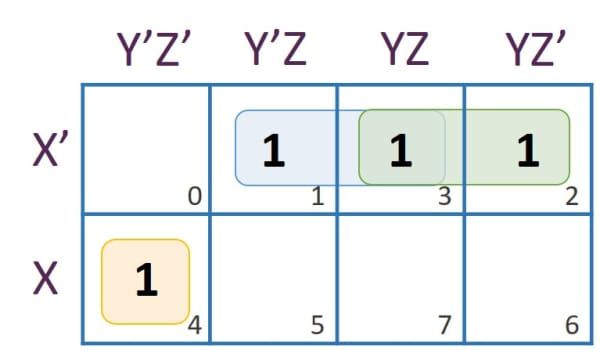From Pair (1,3):
Rows representing the Pair: X'
Columns representing the Pair: Y'Z + YZ = Z
Term Obtained = X'Z

From Pair (3,2):
Rows representing the Pair: X'
Columns representing the Pair: YZ + YZ' = Y
Term Obtained = X'Y

From (4):
Rows representing (4): X
Columns representing (4): Y'Z'
Term Obtained = XY'Z'

Reduced Expression for P = XY'Z' + X'Y + X'Z

K-Map for Q: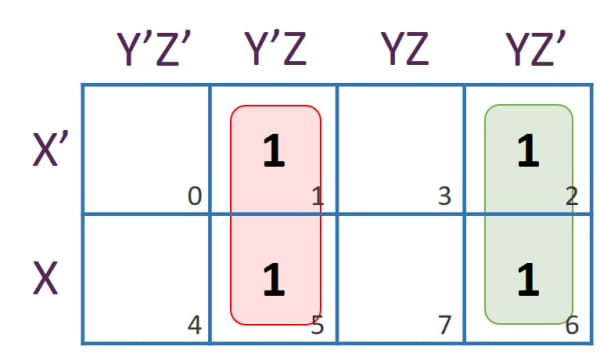From Pair (1,5):
Rows representing the Pair: X' + X = 1
Columns representing the Pair: Y'Z
Term Obtained = Y'Z

From Pair (2,6):
Rows representing the Pair: X' + X = 1
Columns representing the Pair: YZ'
Term Obtained = YZ'

Reduced Expression for Q = Y'Z + YZ'

K-Map for R: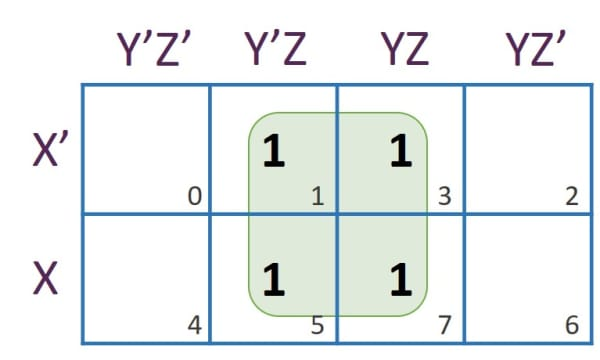Rows representing the Quad: X' + X = 1
Columns representing the Quad: Y'Z + YZ = Z
Term Obtained = Z

Reduced Expression for R = Z

#### Question 50

Convert the following function into its canonical sum of product form:
F(X, Y, Z) = Σ(0,1,5,7)

Canonical sum of product form:
X'Y'Z' + X'Y'Z + XY'Z + XYZ

#### Question 51

Show that dual of P'QR' + PQ'R + P'Q'R is equal to the complement of PQ'R + Q.(P'R' + PR')

Dual of P'QR' + PQ'R + P'Q'R:

(P'+Q+R').(P+Q'+R).(P'+Q'+R)

Complement of PQ'R + Q.(P'R' + PR'):

[PQ'R+Q.(P'R'+PR')]'
= (PQ'R)'.[Q.(P'R'+PR')]'
= (P'+Q+R').[P'QR'+PQR']'
= (P'+Q+R').(P+Q'+R).(P'+Q'+R)

Hence proved.

#### Question 52

What are maxterms? Convert the following function as a product of maxterms:
F(P, Q, R)= (P + Q).(P' + R')

In POS expression, a sum term of n variables in which each of the n variables appear once in either its complement or uncomplement form is known as Max term.

Converting into product of maxterms:
(P + Q).(P' + R')
= (P + Q + RR').(P' + QQ' + R')
= (P + Q + R).(P + Q + R').(P' + Q + R').(P' + Q' + R')

#### Question 53

Obtain the truth table to verify the following expression:
X.(Y + Z)= X.Y + X.Z
Also state this law.

This is Distributive Law.

Truth Table

XYZY+ZX.(Y+Z)X.YX.ZX.Y+X.Z
00000000
00110000
01010000
01110000
10000000
10111011
11011101
11111111

As columns X.(Y+Z) and X.Y+X.Z have same values, hence the expression is proved.

#### Question 54

Given F = A + (B + C).(D' + E)
Find F' and show the relevant working in steps.

``````
F' = [A + (B + C).(D' + E)]'
= A'.[(B + C).(D' + E)]'     [De-Morgan's Law]
= A'.[(B + C)' + (D' + E)']  [De-Morgan's Law]
= A'.[(B'C') + (D''E')]      [De-Morgan's Law]
= A'.(B'C' + DE')            [∵ D'' = D]
= A'B'C' + A'DE'             [Distributive Law]
```
```

#### Question 55

For the given truth table A, B and C are the inputs and X is the output.

ABCX
0001
0010
0100
0111
1001
1010
1101
1110

Write:
(i) Canonical Sum of Product expression (SOP).
(ii) Canonical Product of Sum expression (POS).

ABCXMin
Terms
Max
Terms
0001A'B'C'
0010A+B+C'
0100A+B'+C
0111A'BC
1001AB'C'
1010A'+B+C'
1101ABC'
1110A'+B'+C'

Canonical Sum of Product expression (SOP):
A'B'C' + A'BC + AB'C' + ABC'

Canonical Product of Sum expression (POS):
(A+B+C').(A+B'+C).(A'+B+C').(A'+B'+C')

#### Question 56

Simplify the following expression and convert it into its canonical POS form:
(X.Y + Z)(Y + Z'.X)

Simplifying the expression:

``````

(X.Y + Z)(Y + Z'.X)
= (X + Z).(Y + Z).(Y + Z').(X + Y)
= (XY + YZ + XZ + Z).(Y + Z').(X + Y)
= [XY + YZ + Z(X + 1)].(Y + Z').(X + Y)
= (XY + YZ + Z).(Y + Z').(X + Y)
= (XY + YZ + YZ + XYZ' + YZZ' + ZZ').(X + Y)
= [XY(1 + Z') + YZ + 0 + 0].(X + Y)*
= (XY + YZ).(X + Y)
= XY + XYZ + XY + YZ
= XY(1 + 1 + Z) + YZ
= XY + YZ

```
```

Converting to canonical POS form:

``````

XY + YZ
= Y(X + Z)
= (Y + XX' + ZZ').(X + YY' + Z)
= (X + Y + Z).(X + Y + Z').(X' + Y + Z).(X' + Y + Z').(X + Y + Z).(X + Y' + Z)
= (X + Y + Z).(X + Y + Z').(X' + Y + Z).(X' + Y + Z').(X + Y' + Z)

```
```

#### Question 57

Minimise the following expression. At each step clearly mention the law used.
Y.(A+B').(B+CD)'

``````

Y.(A + B').(B + CD)'
= Y.(A + B').[B'.(CD)']           [De-Morgan's Law]
= Y.(A + B').[B'.(C' + D')]       [De-Morgan's Law]
= (AY + B'Y).(B'C' + B'D')        [Distributive Law]
= AB'C'Y + AB'D'Y + B'C'Y + B'D'Y [Distributive Law]
= B'C'Y(A + 1) + B'D'Y(A + 1)     [Distributive Law]
= B'C'Y + B'D'Y                   [∵ A+1=1]

```
```

#### Question 58

State the distributive law. Verify it using the truth table.

This law states that:

1. A(B + C) = A.B + A.C
2. A + BC = (A + B).(A + C)

Truth Table of first law

ABCB+CA.(B+C)A.BA.CA.B+A.C
00000000
00110000
01010000
01110000
10000000
10111011
11011101
11111111

Truth Table of second law

ABCBCA+BCA+BA+C(A+B).(A+C)
00000000
00100010
01000100
01111111
10001111
10101111
11001111
11111111

#### Question 59

What is the Canonical form of Boolean expression? State the two types of Canonical forms.

A Boolean expression in which each term has all the variables in complement or non-complement form is known as Canonical form of Boolean expression. The two types of Canonical forms are:

1. Sum of Product (SOP)
2. Product of Sum (POS)

#### Question 60

Verify that:
(Z + X)(Z + X' + Y) = (Z + X)(Z + Y)

``````

LHS = (Z + X)(Z + X' + Y)
= Z + X'Z + YZ + XZ + XX' + XY
= Z + XY + Z(Y + X + X') + 0
= Z + XY

RHS = (Z + X)(Z + Y)
= Z + YZ + XZ + XY
= Z(1 + Y + X) + XY
= Z + XY

∴ LHS = RHS
Hence Proved.

```
```

#### Question 61

Prove that F(a, b, c) = π(2, 3, 4, 7) = Σ(0, 1, 5, 6).

F(a, b, c) = π(2, 3, 4, 7)
Reducing using K-Map: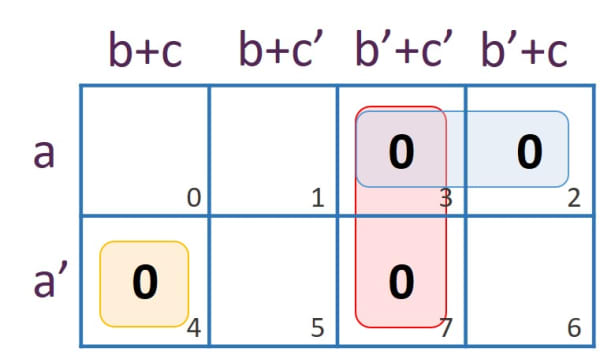From Pair (3,2):
Rows representing the Pair: a
Columns representing the Pair: (b'+c').(b'+c) = b'
Term Obtained = a+b'

From Pair (3,7):
Rows representing the Pair: 1 (Both a and a' cancel each other)
Columns representing the Pair: b'+c'
Term Obtained = b'+c'

From (4):
Row representing (4): a'
Columns representing (4): b+c Term Obtained = a'+b+c

Reduced Expression = (a + b').(b' + c').(a' + b + c)

F(a, b, c) = Σ(0, 1, 5, 6)
Reducing using K-Map: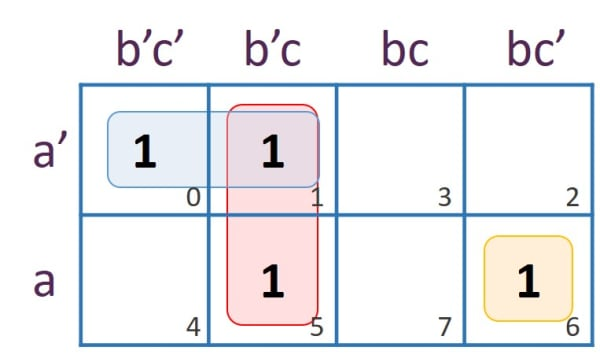From Pair (0,1):
Rows representing the Pair: a'
Columns representing the Pair: b'c' + b'c = b'
Term Obtained = a'b'

From Pair (1,5):
Rows representing the Pair: 1 (Both a and a' cancel each other)
Columns representing the Pair: b'c
Term Obtained = b'c

From (6):
Row representing (6): a
Columns representing (6): bc' Term Obtained = abc'

Reduced Expression = a'b' + b'c + abc'

To prove:
(a + b').(b' + c').(a' + b + c) = a'b' + b'c + abc'

``````

LHS = (a' + b + c).(a + b').(b' + c')
= (aa' + a'b' + ab + bb' + ac + b'c).(b' + c')
= (a'b' + ab + ac + b'c).(b' + c')
= a'b'b' + a'b'c' + abb' + abc' + ab'c + acc' + b'b'c + b'cc'
= a'b' + a'b'c' + 0 + abc' + ab'c + 0 + b'c + 0
= a'b' + a'b'c' + abc' + ab'c + b'c
= a'b'(1 + c') + abc' + b'c(a + 1)
= a'b' + abc' + b'c
= RHS

Hence Proved.

```
```

#### Question 62

State the dual form of the following:
XY'(XY'Z + X + X'Z')